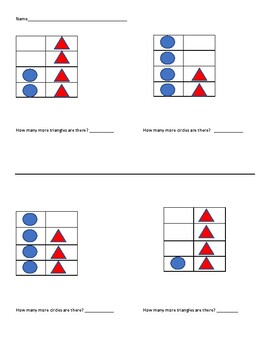# Simple Visual Subtraction by counting the DifferenceSubject
Resource Type
File Type

PDF

(52 KB|2 pages)
Standards
• Product Description
• StandardsNEW

Students will see the shapes lined up to see how many "more" one shape has than another. This is comparing to see the difference. It is another way to look at subtraction.

Fluently add and subtract within 5.
For any number from 1 to 9, find the number that makes 10 when added to the given number, e.g., by using objects or drawings, and record the answer with a drawing or equation.
Decompose numbers less than or equal to 10 into pairs in more than one way, e.g., by using objects or drawings, and record each decomposition by a drawing or equation (e.g., 5 = 2 + 3 and 5 = 4 + 1).
Solve addition and subtraction word problems, and add and subtract within 10, e.g., by using objects or drawings to represent the problem.
Represent addition and subtraction with objects, fingers, mental images, drawings, sounds (e.g., claps), acting out situations, verbal explanations, expressions, or equations.
Total Pages
2 pages
N/A
Teaching Duration
N/A
Report this Resource to TpT
Reported resources will be reviewed by our team. Report this resource to let us know if this resource violates TpT’s content guidelines.